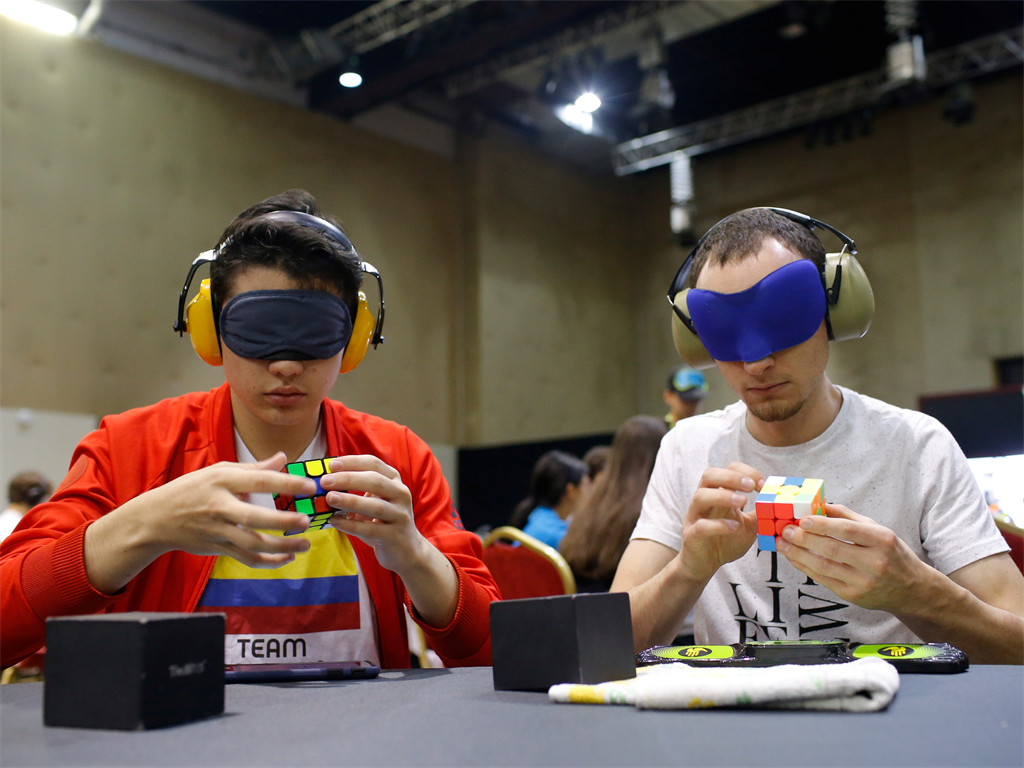Competitors solve Rubik's cubes as they prepare for the world's largest Rubik's Cube championship in Aubervilliers, near Paris, France, July 15, 2017.

• The Rubik's Cube is an iconic puzzle toy.
• But it is mathematically complicated - there are 43 quintillion possible configurations of the Cube.
• Over 30 years after the Cube was invented, a group of mathematicians showed, using a bank of supercomputers at Google, that any cube could be solved in at most 20 moves.

The Rubik's Cube is a classic puzzle toy invented in 1974 by Hungarian architecture and design professor Erno Rubik.

The toy consists of a cube made up of 27 smaller cubes arranged in a 3x3x3 grid with colored stickers on the outer faces of the smaller cubes. A cube starts out in its "solved" configuration with the smaller faces each of the six sides sharing the same color. Each of the six faces of the cube can be rotated freely, moving the smaller cubes around.

The goal of a Rubik's Cube puzzle is to start with some randomized and shuffled messy configuration of the cube and, by rotating the faces, get back to the original solved pattern with each side being a single color.

Actually solving the puzzle is notoriously tricky. It took Erno Rubik himself about a month after inventing the cube to be able to solve it.

Since then, several methods and techniques have been developed for solving a Rubik's Cube, like this basic strategy laid out on the official Rubik's Cube site. Practiced cube-solvers can complete the puzzle in a matter of seconds, with the current world-record holder solving a cube in 4.22 seconds.

Puzzles like the Rubik's Cube are the kind of thing that fascinate mathematicians. The toy's geometrical nature lends itself nicely to mathematical analysis.

There are 18 basic moves that can be applied to a Rubik's Cube: rotating one of the six faces - front, back, up, down, left, or right - either 90° clockwise, 90° counterclockwise, or 180°. Any solution to a particular Rubik's Cube configuration, then, can be thought of as the list of basic moves needed to return that configuration to the starting solved state.

One immediate and obvious question, dating back to the original invention of the cube, is, given a particular configuration of a cube, what's the smallest number of moves needed to solve the puzzle? Relatedly, what is the smallest number of moves needed to solve any configuration of the Rubik's Cube, a number that cube aficionados refer to as "God's number?"

As Erno Rubik put it in a recent interview with Business Insider, this question is "connected with the mathematical problems of the cube."

Amazingly, it took 36 years after the invention of the toy to come up with an answer. In 2010, a group of mathematicians and computer programmers proved that any Rubik's Cube can be solved in, at most, 20 moves.

One reason it took so long to answer such an apparently straightforward question is the surprising complexity of the Rubik's Cube. An analysis of all the possible permutations of where the smaller constituent cubes (often called "cubies") can end up shows that there are about 43 quintillion - 43,000,000,000,000,000,000 - possible configurations of the Rubik's Cube.

Going through and trying to find the shortest solution for every single one of those configurations, then, is essentially impossible. The key to answering a question like finding the smallest number of moves to solve any configuration is to take advantage of the relationships between different configurations.

In 1995, mathematician Michael Reid found a Rubik's Cube configuration called a "superflip" and proved that it required at least 20 moves to solve. That sets a lower limit on what God's Number could be. The remaining question, then, is whether or not there are any cubes that need more than 20 steps to solve.

Over the decades, various upper bounds were proven. An early mathematical analyst of the cube, Morwen Thistlethwaite, was able to prove that any cube could be solved in at most 52 moves.

Computer programmer Tomas Rokicki came up with a strategy for finding relatively short solutions for Rubik's Cube configurations. The strategy was based on earlier work developed by mathematician Herbert Kociemba that broke solving a cube into two steps, based on a special set of about 19.5 billion partially-solved configurations that are known to have relatively short solutions. Step one, then, is to move the cube into one of those configurations, and step two is to use the short solution for that partially solved configuration.

Kociemba's algorithm is the basis of many computer-operated robotic cube solvers, like the one in the video below:

Previous work using this strategy showed that it would take at most 30 moves to solve any cube: Each of the configurations in the smaller special set take at most 18 steps to solve, and any cube configuration takes at most 12 steps to get to one of the special states.

Rokicki took the strategy a step further by grouping together configurations using the special partially-solved configuration set. This essentially meant being able to solve 19.5 billion configurations at once. As Rokicki and his colleagues put it on their site, they "partitioned the positions into 2,217,093,120 sets of 19,508,428,800 positions each."

So, Rokicki's strategy meant having to work with about 2.2 billion problems, rather than the original 43 quintillion. That remained a daunting computational task, but in 2010, Rokicki and his colleagues used a bank of supercomputers at Google to finally show that 20 was in fact the magic number.

Of course, the fact that answering this question took over three decades, required some deep mathematics, and needed a set of supercomputers to finally find out the answer suggests that this may not be the most practical approach to solving a cube for the casual hobbyist. But it is the kind of problem that mathematicians love playing around with.